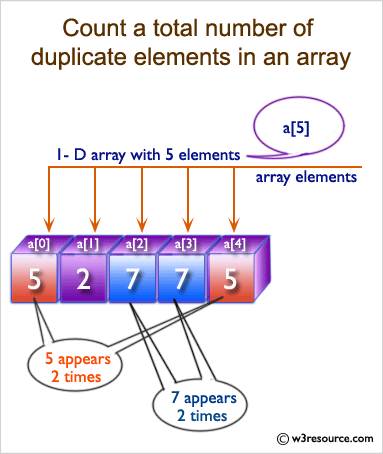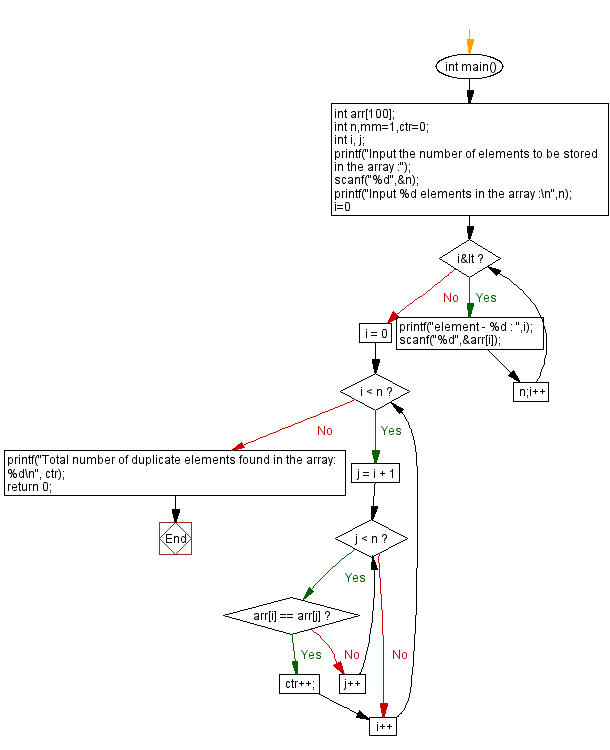﻿ C : Count the total number of duplicate elements in an array

# C Exercises: Count the total number of duplicate elements in an array

## C Array: Exercise-5 with Solution

Write a program in C to count the total number of duplicate elements in an array.

Visual Presentation:Pseudo code to count the total number of duplicate elements in an array:

• Initialize a variable 'count' to 0.
• Read the array size, 'n'.
• Declare an integer array of size 'n'.
• Loop through each element of the array from index 0 to n-2: a. Loop through the remaining elements of the array from index i+1 to n-1: i. If the current element and any of the remaining elements are equal, increment the 'count' variable and break out of the inner loop.
• Print the value of 'count' as the total number of duplicate elements in the array.
• End

Sample Solution:

C Code:

``````#include <stdio.h>
// Main function
int main()
{
int arr;  // Declare an array of size 100 to store integer values
int n, mm = 1, ctr = 0;  // Declare variables to store array size, mm (unused), and duplicate counter
int i, j;  // Declare loop counters
// Prompt the user to input the number of elements to be stored in the array
printf("Input the number of elements to be stored in the array :");
scanf("%d", &n);
// Prompt the user to input n elements into the array
printf("Input %d elements in the array :\n", n);
for (i = 0; i < n; i++)
{
printf("element - %d : ", i);
scanf("%d", &arr[i]);  // Read the input and store it in the array
}
// Check for duplicate elements in the array using nested loops
for (i = 0; i < n; i++)
{
for (j = i + 1; j < n; j++)
{
if (arr[i] == arr[j])
{
ctr++;  // Increment the duplicate counter if a duplicate is found
break;  // Exit the inner loop as soon as a duplicate is found
}
}
}
// Display the total number of duplicate elements found in the array
printf("Total number of duplicate elements found in the array: %d\n", ctr);
return 0;  // Return 0 to indicate successful execution
}
```
```

Sample Output:

```Input the number of elements to be stored in the array :5
Input 5 elements in the array :
element - 0 : 1
element - 1 : 1
element - 2 : 2
element - 3 : 3
element - 4 : 3
Total number of duplicate elements found in the array: 2
```

Flowchart:C Programming Code Editor:

What is the difficulty level of this exercise?

Test your Programming skills with w3resource's quiz.

﻿